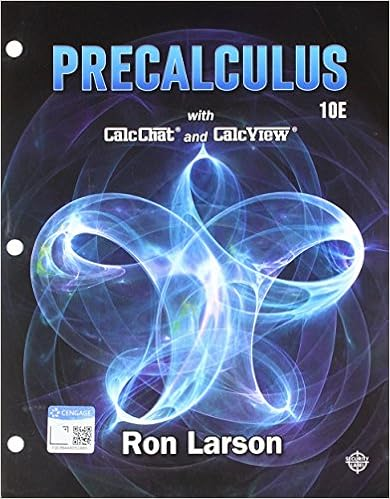# Exercise 1: Find the derivative of the functions below at...

• Test Prep
• nibbles360
• 5
• 100% (2) 2 out of 2 people found this document helpful

This preview shows page 1 - 3 out of 5 pages.

##### We have textbook solutions for you!
The document you are viewing contains questions related to this textbook.The document you are viewing contains questions related to this textbook.
Chapter 6 / Exercise 22
Precalculus
Hostetler/LarsonExpert Verified
Math 2011-Introduction to Multivariable Calculus (Edited by Dr. Hon-Ming HO) Practice Exercises 10: Gradient Vectors and Directional Derivatives Exercise 1: Find the derivative of the functions below at point ? 0 (? 0 , ? 0 ) in the specified direction of ? . ? .
Practice Exercises 10 (edited by Dr. Hon-Ming HO)-page 1 c) Function ℎ(?, ?, ?) = cos(??) + ? ?? + ln|??| at point ? 0 (1 ,0 , 0.5) in the direction of ? ⃑ =< 1 ,2 ,2 > . Solution to Exercise 1: We are required to find directional derivative (𝐷 𝑢 ⃑⃑ ?)(? 0 ) at the specific point in the given direction. There are two ways to find the desired directional derivatives for three parts above. The 1 st way is to apply the limit definition of directional derivative . Assume that 𝒖 ⃑⃑⃑ is a unit vector . Recall the limit definitions of directional derivatives. 2 − variable version: (𝐷 𝑢 ⃑⃑ ?)(? 0 ) = (𝐷 <𝑢 1 ,𝑢 2 > ?)(? 0 , ? 0 ) = lim ℎ→0 ?(? 0 + ℎ? 1 , ? 0 + ℎ? 2 ) − ?(? 0 , ? 0 ) . 3 − variable version: (𝐷 𝑢 ⃑⃑ ?)(? 0 ) = (𝐷 <𝑢 1 ,𝑢 2 ,𝑢 3 > ?)(? 0 , ? 0 , ? 0 ) = lim ℎ→0 ?(? 0 + ℎ? 1 , ? 0 + ℎ? 2 , ? 0 + ℎ? 3 ) − ?(? 0 , ? 0 , ? 0 ) . Part a) (the 1 st method): 1) Things to do: Normalize the given vector ? . In this example, since the given vector ? ⃑ =< 4 ,3 > is not a unit vector, to compute the desired directional derivative, we first make it unit vector by dividing it by its length. ? ⃑⃑ = ? |? ⃑| = < 4 ,3 > √4 2 + 3 2 =< 4 5 , 3 5 > . 2) Things to do: Plug the components of the unit vector in step 1) into the working formulas of ? to compute difference quotient. In part a), we obtain the following difference quotient. ?(? 0 + ℎ? 1 , ? 0 + ℎ? 2 ) − ?(? 0 , ? 0 ) = ? (5 + ℎ ( 4 5 ) , 5 + ℎ ( 3 5 )) − ?(5,5) = (5 + ℎ ( 4 5 )) (5 + ℎ ( 3 5 )) − (5)(5) ,
##### We have textbook solutions for you!
The document you are viewing contains questions related to this textbook.The document you are viewing contains questions related to this textbook.
Chapter 6 / Exercise 22
Precalculus
Hostetler/LarsonExpert Verified
Practice Exercises 10 (edited by Dr. Hon-Ming HO)-page 2 = 25 + 4ℎ + 3ℎ + ℎ 2 ( 12 25 ) − 25 = 7ℎ + ℎ 2 ( 12 25 ) = 7 + ℎ ( 12 25 ) . 3) Things to do: Take limit of the expression obtained in step 2) by letting ℎ → 0 . In part a), we obtain (𝐷 𝑢 ⃑⃑ ?)(? 0 ) = (𝐷 < 4 5 , 3 5 > ?) (5 ,5) = lim ℎ→0 7 + ℎ ( 12 25 ) = 7 . The 2 nd way is to apply the following theorem: Theorem (using gradient to find directional derivatives): If ? = ?(? , ?) is differentiable at ? 0 (? 0 , ? 0 ) and ? ⃑ =< ? 1 , ? 2 > is a unit vector, then the directional derivative of ? at ? 0 in the direction of ? is given by (𝐷 𝑢 ?)(? 0 ) = ? ⃑ ⦁ (𝛻?)(? 0 ) . Part a) (the 2 nd method): 1) Things to do: Normalize the given vector ? . In this example, since the given vector ? ⃑ =< 4 ,3 > is not a unit vector, to compute the desired directional derivative, we first make it unit vector by dividing it by its length. ? ⃑⃑ = ? |? ⃑| = < 4 ,3 > √4 2 + 3 2 =< 4 5 , 3 5 > .
•••